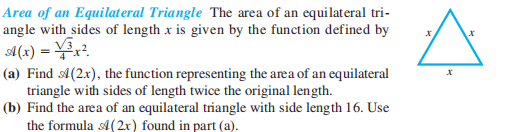### Still have math questions?

Algebra
QuestionArea of an Equilateral Triangle The area of an equilateral tri- angle with sides of length $$x$$ is given by the function defined by $$A ( x ) = \frac { \sqrt { 3 } } { 4 } x ^ { 2 }$$ .

(a) Find $$A ( 2 x )$$ , the function representing the area of an equilateral triangle with sides of length twice the original length.

(b) Find the area of an equilateral triangle with side length $$16$$ . Use the formula $$A ( 2 x )$$ found in part (a).

$$\text { (a) } A ( 2 x ) = \sqrt { 3 } x ^ { 2 } \quad \text { (b) } 64 \sqrt { 3 } \text { square units }$$